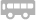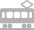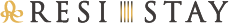## 御碗花见

「お碗」 京都之美学 随处可寻

【碗】之深度逾于器，同时兼具保温性 这里如同【碗】一般，洋溢着热情以迎接客人您的到来，只为演绎一段美好时光于您心间。

##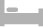房间介绍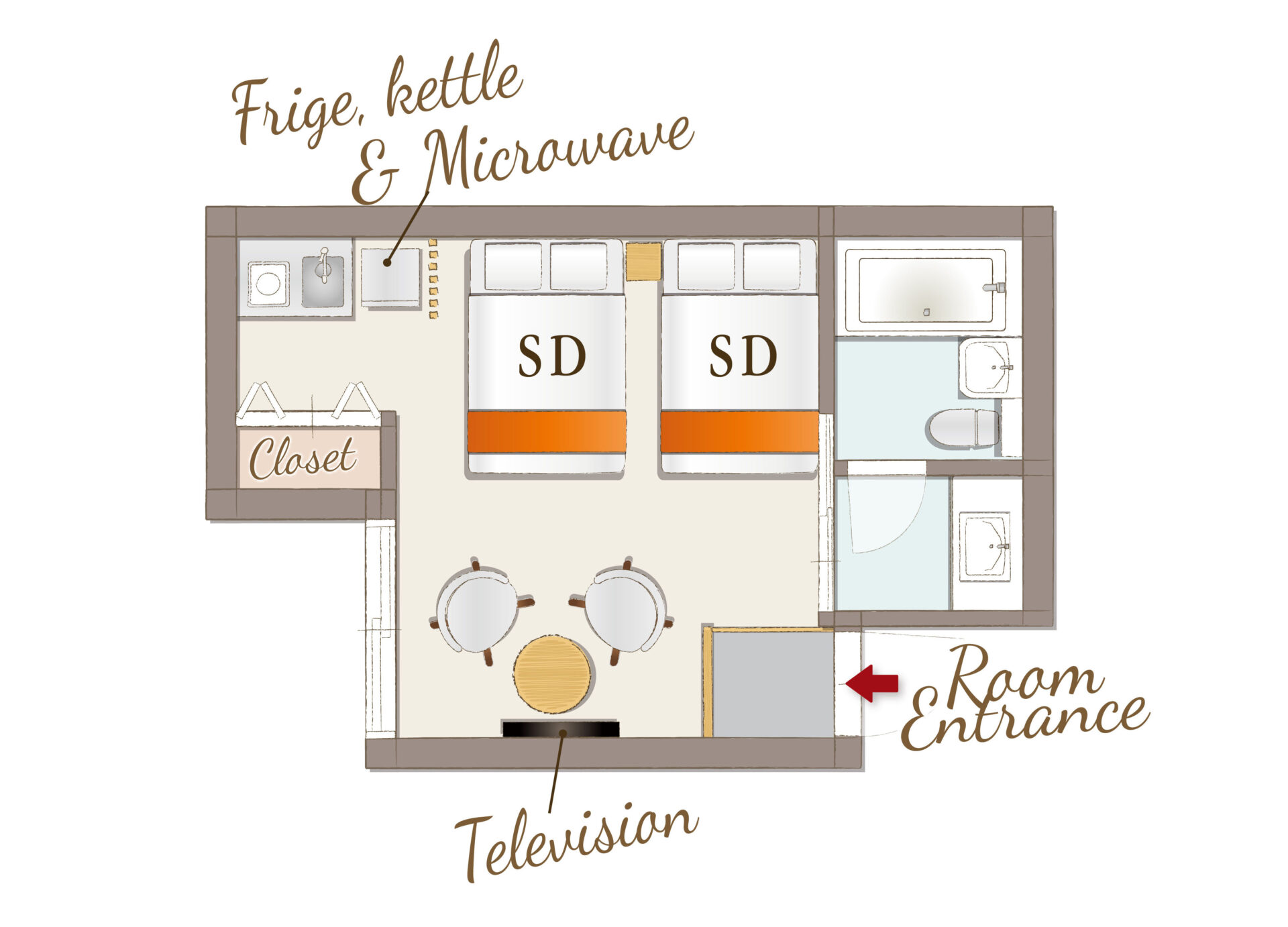## Standard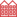公寓式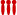可供4位以上入住

[可容纳人数 : 4 位]
・半双人床(幅 1,200mm)× 2张

• 温水便座
• 浴缸
• 吹风机
• 空调
• 保险柜
• TV
• AppleTV/androidTV
• USB充电插口
• Wi-Fi
• 冰箱
• 微波炉
• 电水壶
• 電磁爐
• 衣架
• 电熨斗
• 壁橱
• 吸尘器
• 禁烟

• 洗发水
• 护发素
• 沐浴液
• 洗脸毛巾
• 浴巾
• 浴室防滑垫
• 牙刷
• 洗手液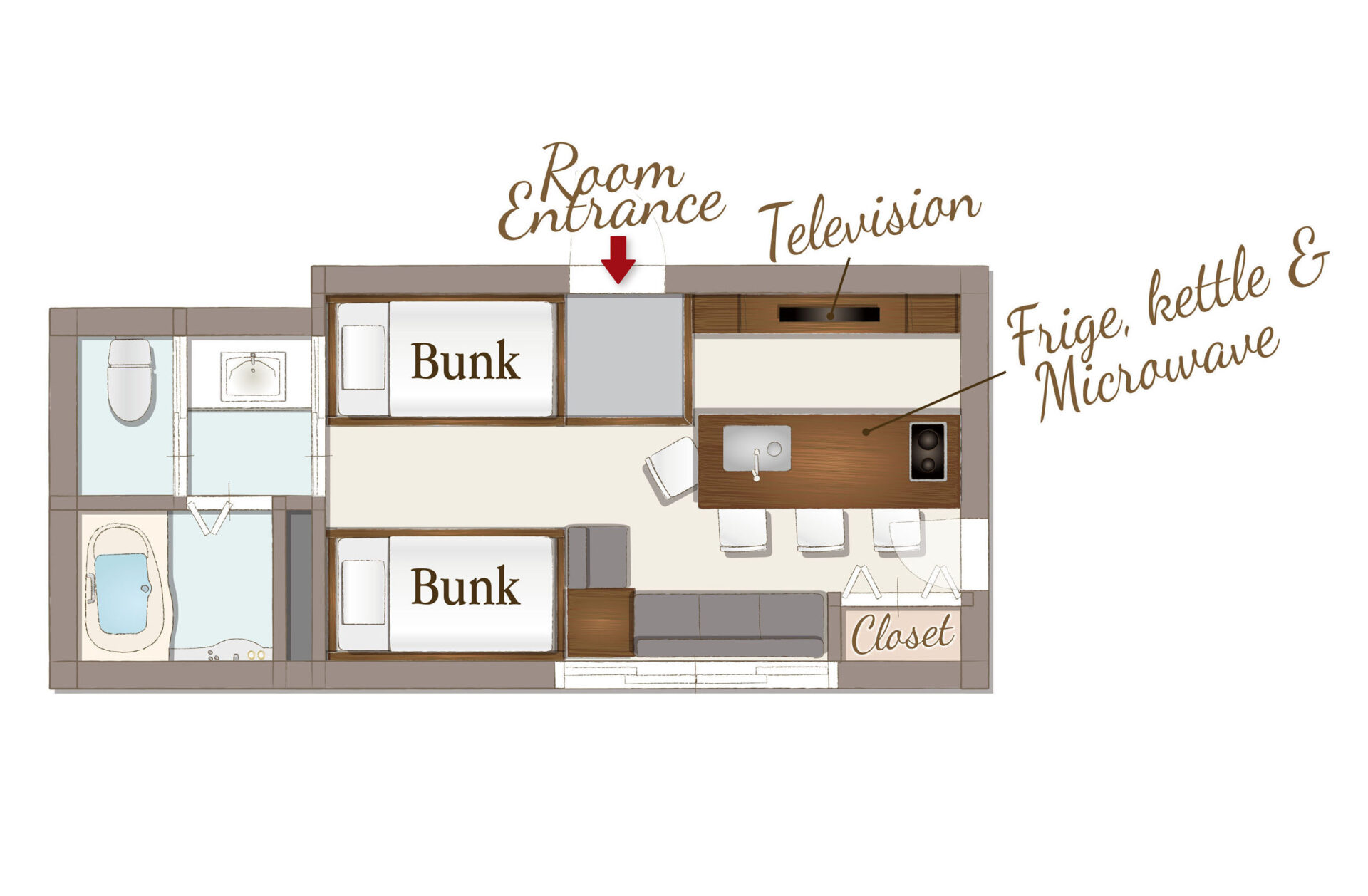## Party Room公寓式可供4位以上入住

[可容纳人数 : 4 位]
・双层床(幅 1,000mm)×2张

• 温水便座
• 浴缸
• 吹风机
• 空调
• 保险柜
• TV
• AppleTV/androidTV
• USB充电插口
• Wi-Fi
• 冰箱
• 微波炉
• 电水壶
• 電磁爐
• 烹调器具
• 电饭煲
• 餐具
• 衣架
• 电熨斗
• 壁橱
• 吸尘器
• 禁烟

• 洗发水
• 护发素
• 沐浴液
• 洗脸毛巾
• 浴巾
• 浴室防滑垫
• 拖鞋
• 化妆棉
• 牙刷
• 化妆棉
• 沐浴剂
• 便笺/笔
• 咖啡/茶/绿茶
• 矿泉水
• 洗手液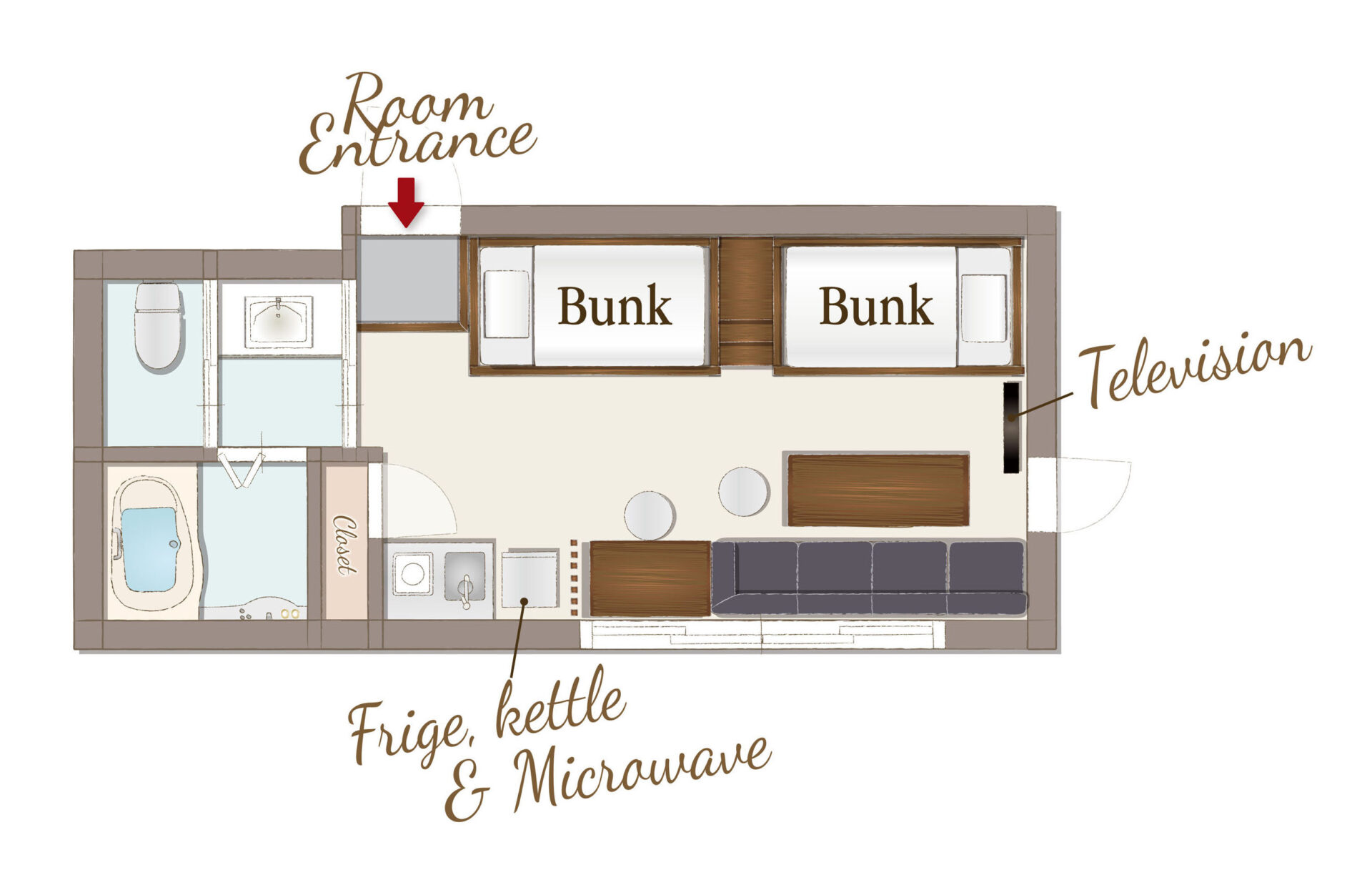## Family A公寓式可供4位以上入住

[可容纳人数 : 4 位]
・双层床(幅 1,000mm)×2张

• 温水便座
• 浴缸
• 吹风机
• 空调
• 保险柜
• TV
• AppleTV/androidTV
• USB充电插口
• Wi-Fi
• 冰箱
• 微波炉
• 电水壶
• 電磁爐
• 烹调器具
• 餐具
• 衣架
• 电熨斗
• 壁橱
• 吸尘器
• 禁烟

• 洗发水
• 护发素
• 沐浴液
• 洗脸毛巾
• 浴巾
• 浴室防滑垫
• 拖鞋
• 化妆棉
• 牙刷
• 化妆棉
• 沐浴剂
• 便笺/笔
• 咖啡/茶/绿茶
• 矿泉水
• 洗手液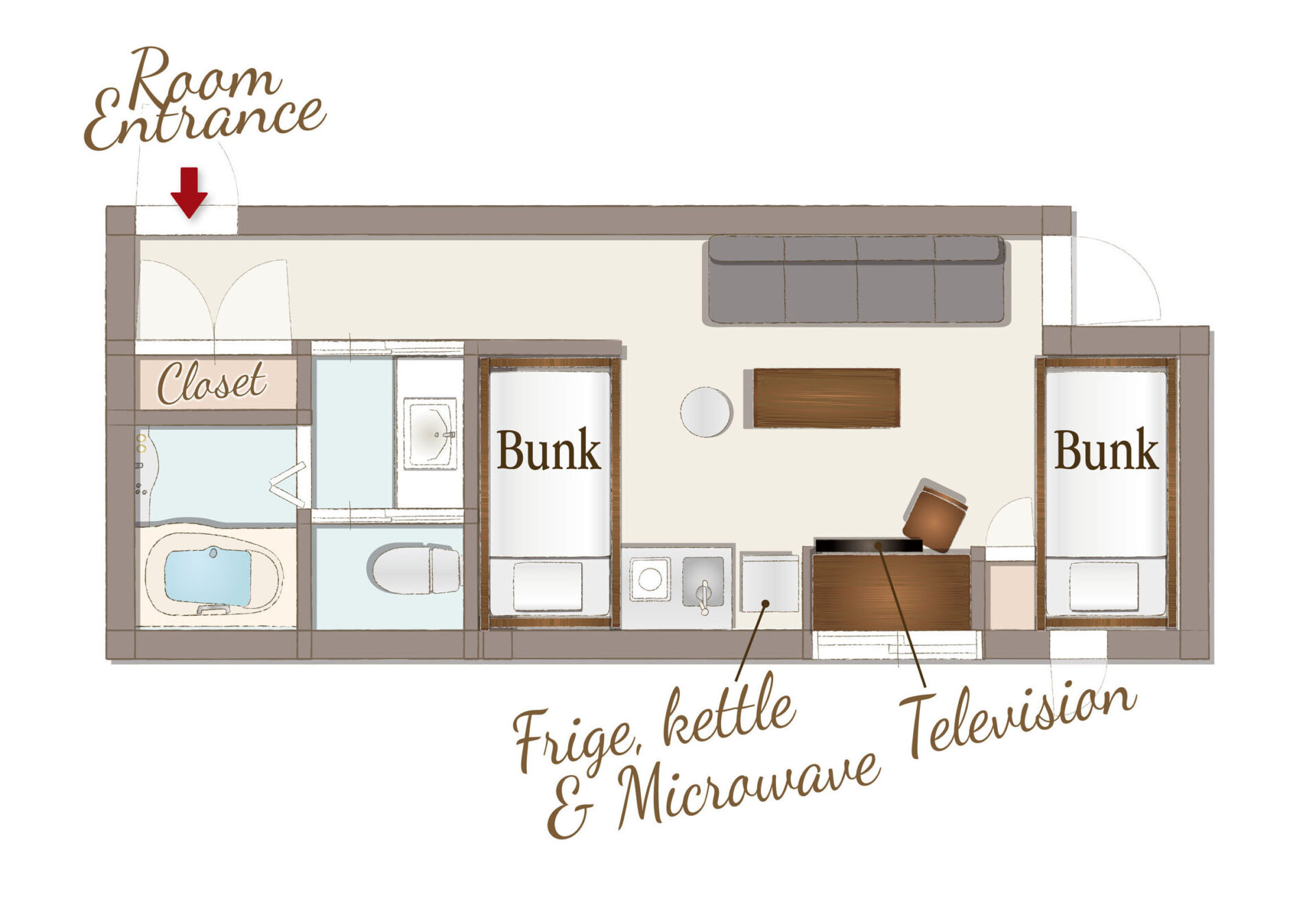## Family B公寓式可供4位以上入住

[可容纳人数 : 4 位]
・双层床(幅 1,000mm)×2张

• 温水便座
• 浴缸
• 吹风机
• 空调
• 保险柜
• TV
• AppleTV/androidTV
• USB充电插口
• Wi-Fi
• 冰箱
• 微波炉
• 电水壶
• 電磁爐
• 烹调器具
• 餐具
• 衣架
• 电熨斗
• 壁橱
• 吸尘器
• 禁烟

• 洗发水
• 护发素
• 沐浴液
• 洗脸毛巾
• 浴巾
• 浴室防滑垫
• 拖鞋
• 化妆棉
• 牙刷
• 化妆棉
• 沐浴剂
• 便笺/笔
• 咖啡/茶/绿茶
• 矿泉水
• 洗手液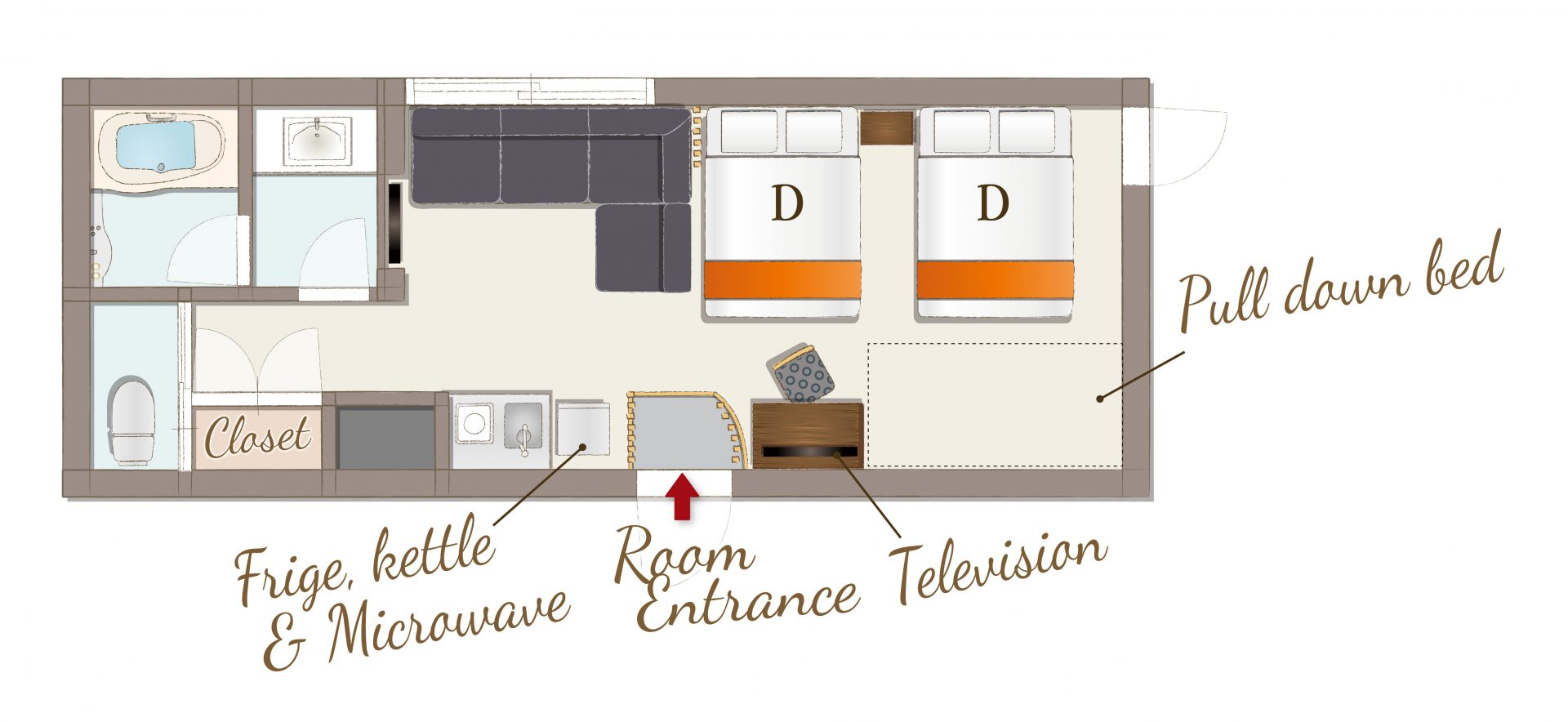## Superior Double Beds公寓式可供4位以上入住

[可容纳人数 : 5 位]
・双人床(幅 1,400mm) × 2张
・折叠床(幅 1,000mm) × 1张

• 温水便座
• 浴缸
• 吹风机
• 空调
• 保险柜
• TV
• AppleTV/androidTV
• USB充电插口
• Wi-Fi
• 冰箱
• 微波炉
• 电水壶
• 電磁爐
• 烹调器具
• 餐具
• 衣架
• 电熨斗
• 壁橱
• 吸尘器
• 禁烟

• 洗发水
• 护发素
• 沐浴液
• 洗脸毛巾
• 浴巾
• 浴室防滑垫
• 拖鞋
• 化妆棉
• 牙刷
• 化妆棉
• 沐浴剂
• 便笺/笔
• 咖啡/茶/绿茶
• 矿泉水
• 洗手液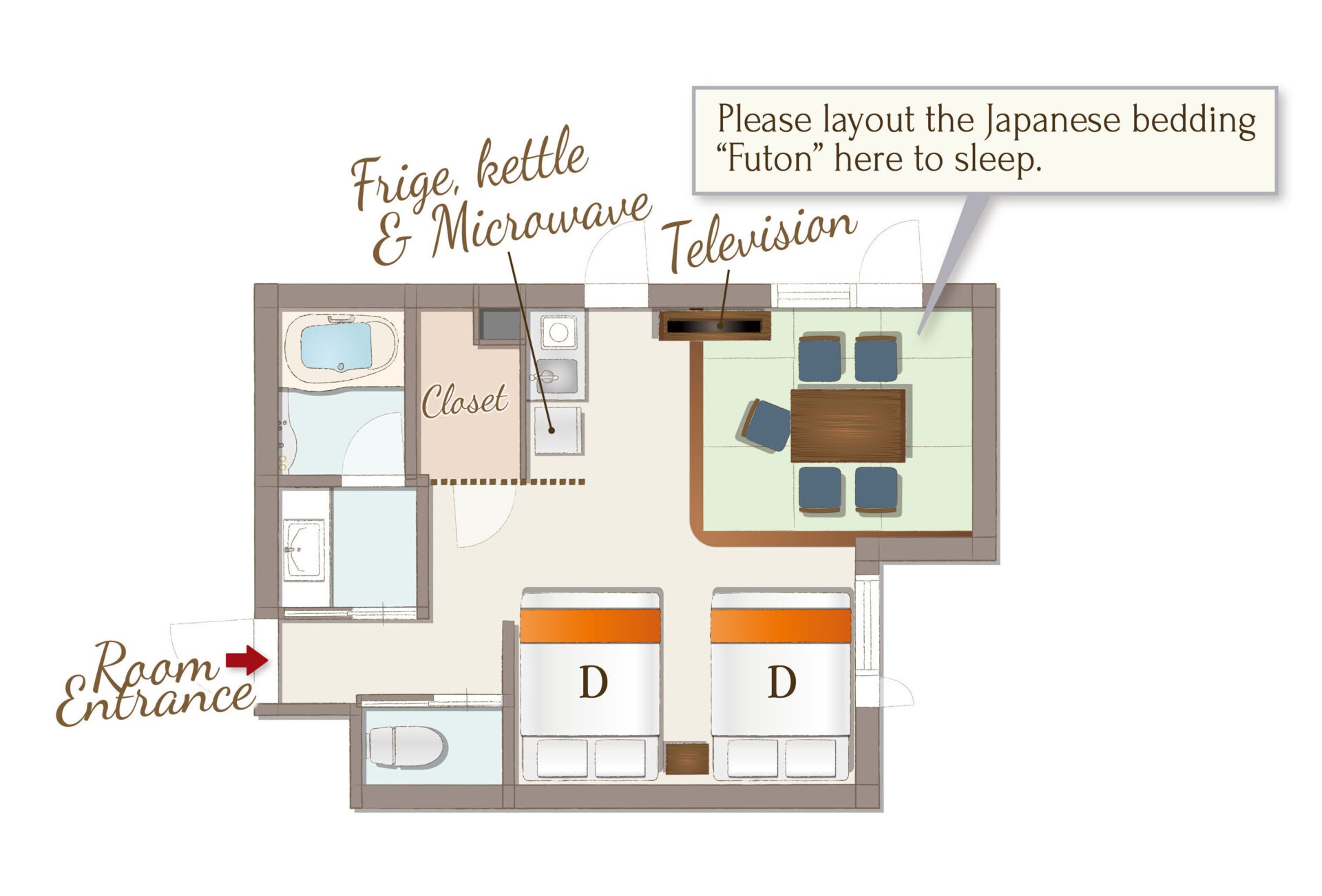## Deluxe Japanese公寓式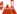配有日式客房的住宿设施可供4位以上入住

[可容纳人数 : 5 位]
・双人床(幅 1,400mm) × 2张
・日式蒲团(幅 970mm) × 1张

• 温水便座
• 浴缸
• 吹风机
• 空调
• 保险柜
• TV
• AppleTV/androidTV
• USB充电插口
• Wi-Fi
• 冰箱
• 微波炉
• 电水壶
• 電磁爐
• 烹调器具
• 餐具
• 衣架
• 电熨斗
• 壁橱
• 吸尘器
• 禁烟

• 洗发水
• 护发素
• 沐浴液
• 洗脸毛巾
• 浴巾
• 浴室防滑垫
• 拖鞋
• 化妆棉
• 牙刷
• 化妆棉
• 沐浴剂
• 便笺/笔
• 咖啡/茶/绿茶
• 矿泉水
• 洗手液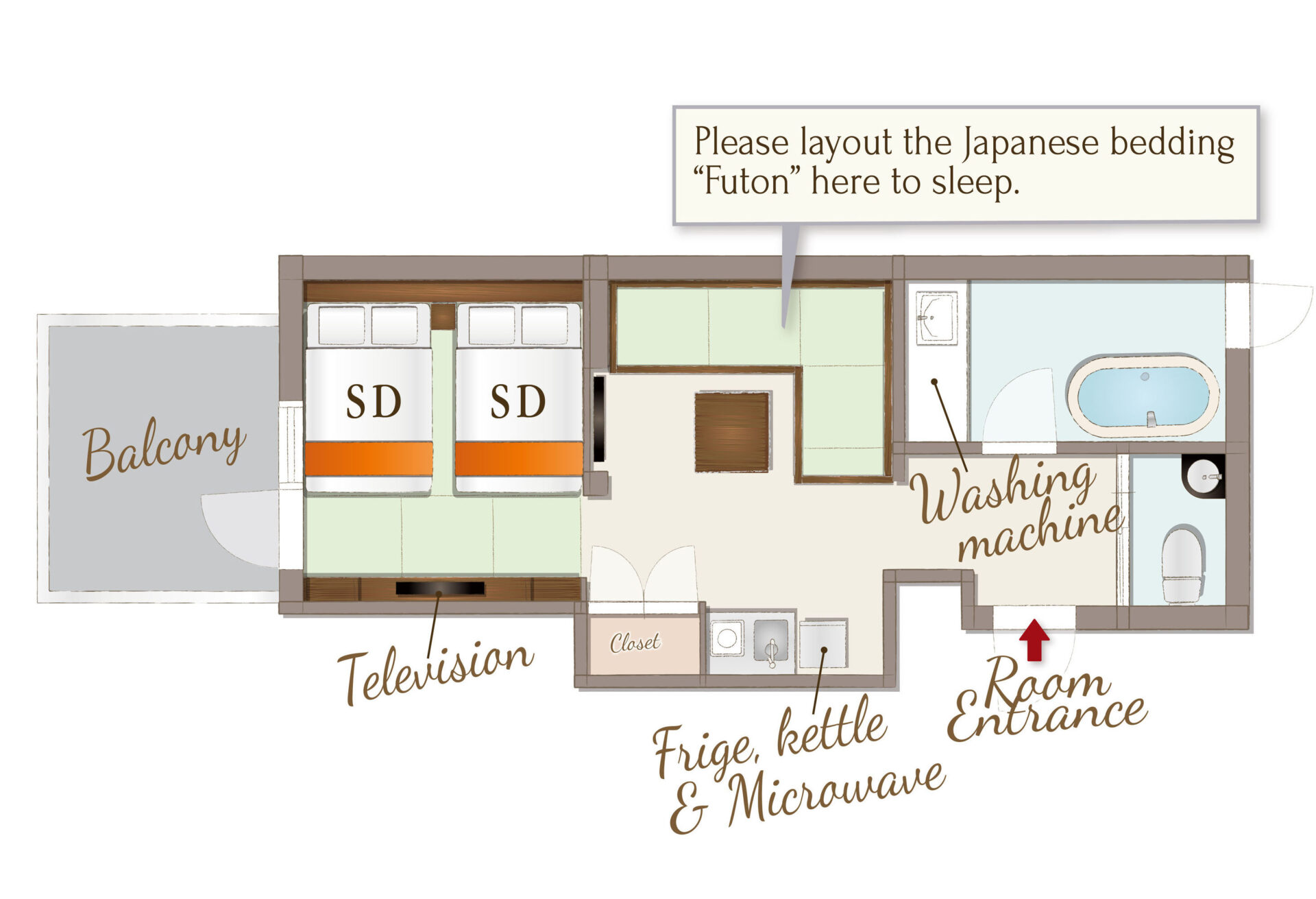## Balcony Junior Suite公寓式可供4位以上入住

[可容纳人数 : 5 位]
・半双人床(幅 1,200mm)× 2张
・日式蒲团(幅 970mm) × 1张

• 温水便座
• 浴缸
• 吹风机
• 空调
• 保险柜
• TV
• AppleTV/androidTV
• USB充电插口
• Wi-Fi
• 冰箱
• 微波炉
• 电水壶
• 電磁爐
• 烹调器具
• 餐具
• 洗衣机
• 衣架
• 电熨斗
• 壁橱
• 吸尘器
• 禁烟

• 洗发水
• 护发素
• 沐浴液
• 洗脸毛巾
• 浴巾
• 浴室防滑垫
• 拖鞋
• 化妆棉
• 牙刷
• 化妆棉
• 沐浴剂
• 便笺/笔
• 咖啡/茶/绿茶
• 矿泉水
• 洗手液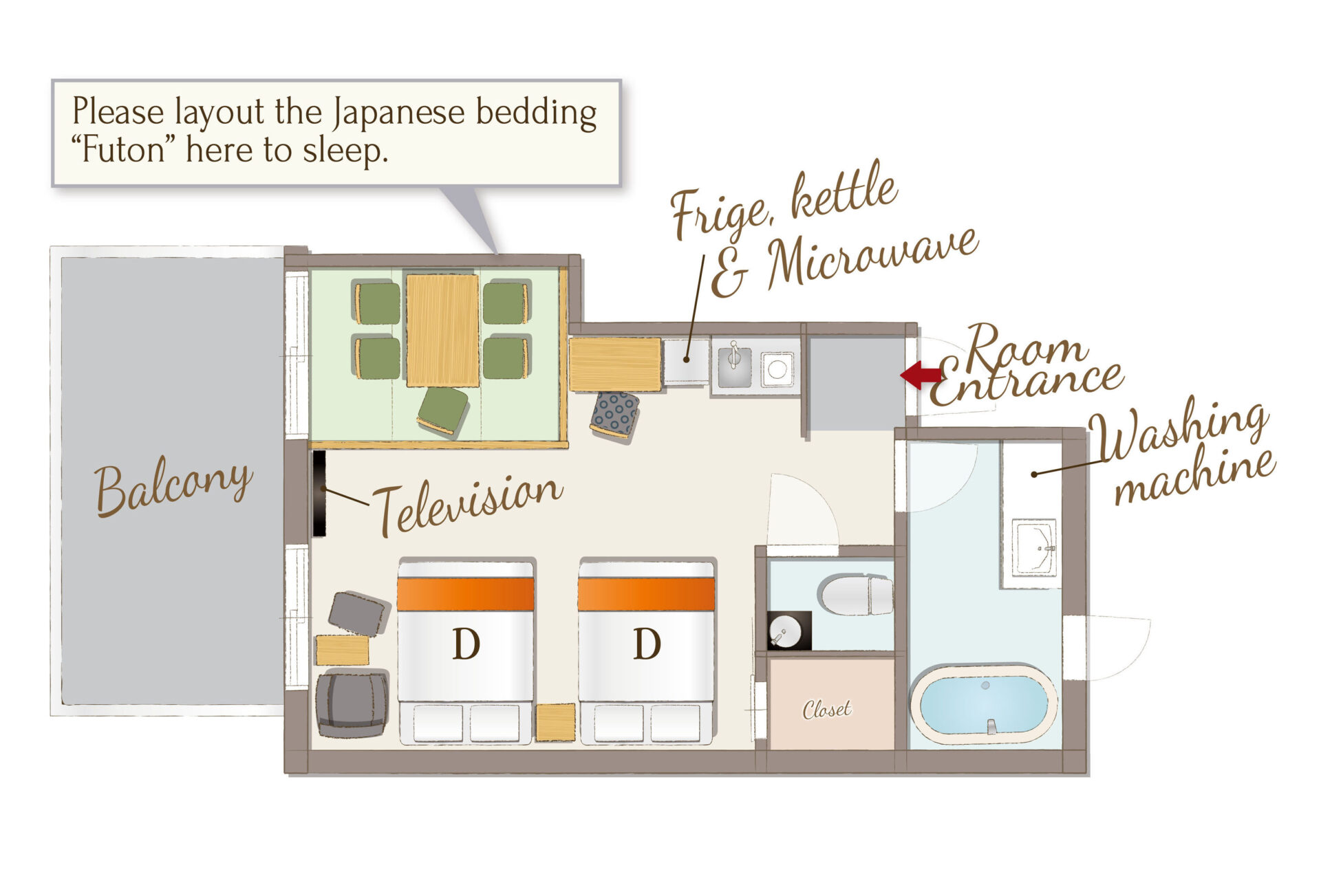## Balcony Family公寓式配有日式客房的住宿设施可供4位以上入住

[可容纳人数 : 5 位]
・双人床(幅 1,400mm) × 2张
・日式蒲团(幅 970mm) × 1张

• 温水便座
• 浴缸
• 吹风机
• 空调
• 保险柜
• TV
• AppleTV/androidTV
• USB充电插口
• Wi-Fi
• 冰箱
• 微波炉
• 电水壶
• 電磁爐
• 烹调器具
• 餐具
• 洗衣机
• 衣架
• 电熨斗
• 壁橱
• 吸尘器
• 禁烟

• 洗发水
• 护发素
• 沐浴液
• 洗脸毛巾
• 浴巾
• 浴室防滑垫
• 拖鞋
• 化妆棉
• 牙刷
• 化妆棉
• 沐浴剂
• 便笺/笔
• 咖啡/茶/绿茶
• 矿泉水
• 洗手液

##交通